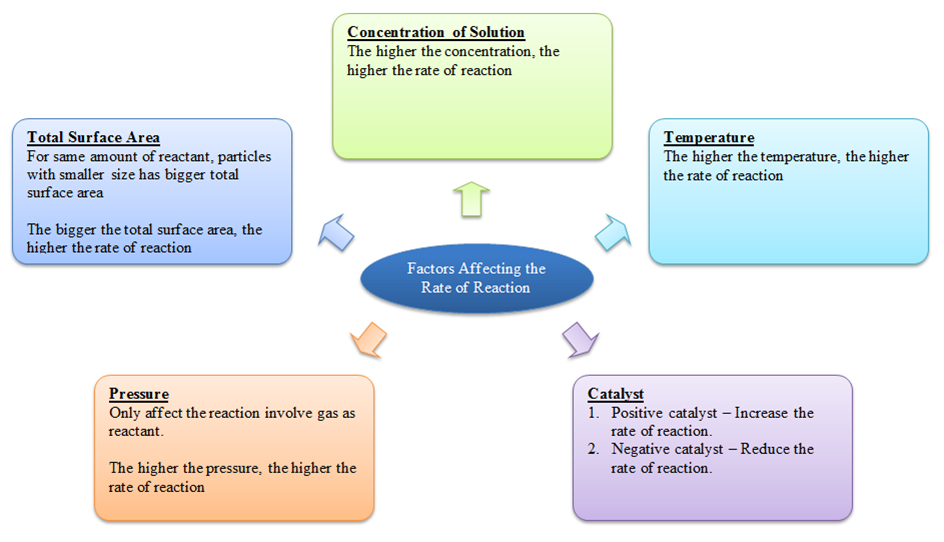# Factors Influencing the Rate of Reaction

• The rate of reaction is governed by number of factors. Those having appreciable effect are described below:

a) Nature of reactants

• Reactions involving polar and ionic substances including the proton-transfer reactions are usually very fast.
• On the other hand, the reactions in which bonds are rearranged, or electrons transferred, are slow.
• Oxidation- reduction reactions, which involve transfer of electrons, are also slow as compared to the ionic reactions.
• Substitution reactions are relatively much slower.
• For example, the reaction between H3O+ and OH is extremely fast (k = 1011 L mol-1 s-1).
• The fastness of the reaction may be seen from the fact that if 0.1M solutions of a strong acid and strong base are mixed, 99% of the reaction will be over within 10-6s.
• The rate constant for the reaction

CH3COOCH3 + H2O      ⇌ (H+)      CH3COOH + CH3OH

Which involves breaking and reformation of bonds is of the order 10-2 s-1.

b) Temperature

• The reaction rate generally increases with a rise in temperature.
• The effect of temperature is commonly described in terms of temperature coefficient of the reaction.
• The temperature coefficient is defined as the ratio of the rate constants at two temperatures differing by 100C, usually from 25 to 350C.
• The temperature coefficients for most of the reactions lie between 2 and 3, i.e., the reaction rates increase by a factor of 2 to 3, for every 10 degree rise in temperature.

c) Concentration of the reactants

• The rate of reaction increases with an increase in the concentration of the reactants.
• This is due to an increased probability of the molecular collisions at higher concentrations.
• The law of mass action explains the effect of concentration on the rate of reaction.
• According to this law, if the reaction proceeds in a single step as indicated by the balanced chemical equation, then the rate of a chemical reaction is proportional to the product of the concentrations of the reacting substances, each raised to the power equal to the stoichiometric coefficient occurring in the balanced chemical equation.Image source: minichemistry

d) Catalyst

• Catalyst is a substance that changes the reaction rate without undergoing any permanent change in its chemical behavior.
• It was given by Ostwald in 1895.
• Broadly, there are two types of catalyst; positive and negative catalysts.
• The positive catalysts increase the rate of reaction, while negative catalysts decrease the reaction rate.
• A positive catalyst is believed to provide the reaction with an alternative pathway involving lower activation energy.
• The lowering of activation energy by the catalyst permits more and more molecules to take part in the chemical reaction, leading to an increase in the rate of reaction.Image source: byjus

e) Physical state of the reactants

• The reaction rate also depends upon the ease with which the reactants are brought in contact with each other.
• Thus, ionic reactions are faster in the high dielectric constant solvents than in the solid state.
• A powdered substance tends to react faster than the material in the lump form.
• For example, coal (lump form) burns at a normal rate when ignited, but if the powdered coal is ignited, it burns in an explosive way.
• This happens due to larger surface area of the coal in the powdered form as compared to that in the lump form.

f) Pressure

• Pressure also has a significant effect on the rate of certain reactions.
• Increase in pressure e.g., by hammering can cause many reactions going in an explosive way.

References: